# RD Sharma Solutions for Class 10 Maths Chapter 16 Surface Areas And Volumes Exercise 16.2

In our day-to-day lives, we come across various solids which are combinations of two or more such solids. Finding the surface areas and volumes of combinations of solids is majorly tested in this exercise. The RD Sharma Solutions Class 10 can be utilised by the students to clarify any doubts pertaining to this chapter. Also, students can also get the RD Sharma Solutions for Class 10 Maths Chapter 16 Surface Areas And Volumes Exercise 16.2 PDF provided below.

## RD Sharma Solutions for Class 10 Maths Chapter 16 Surface Areas And Volumes Exercise 16.2 Download PDF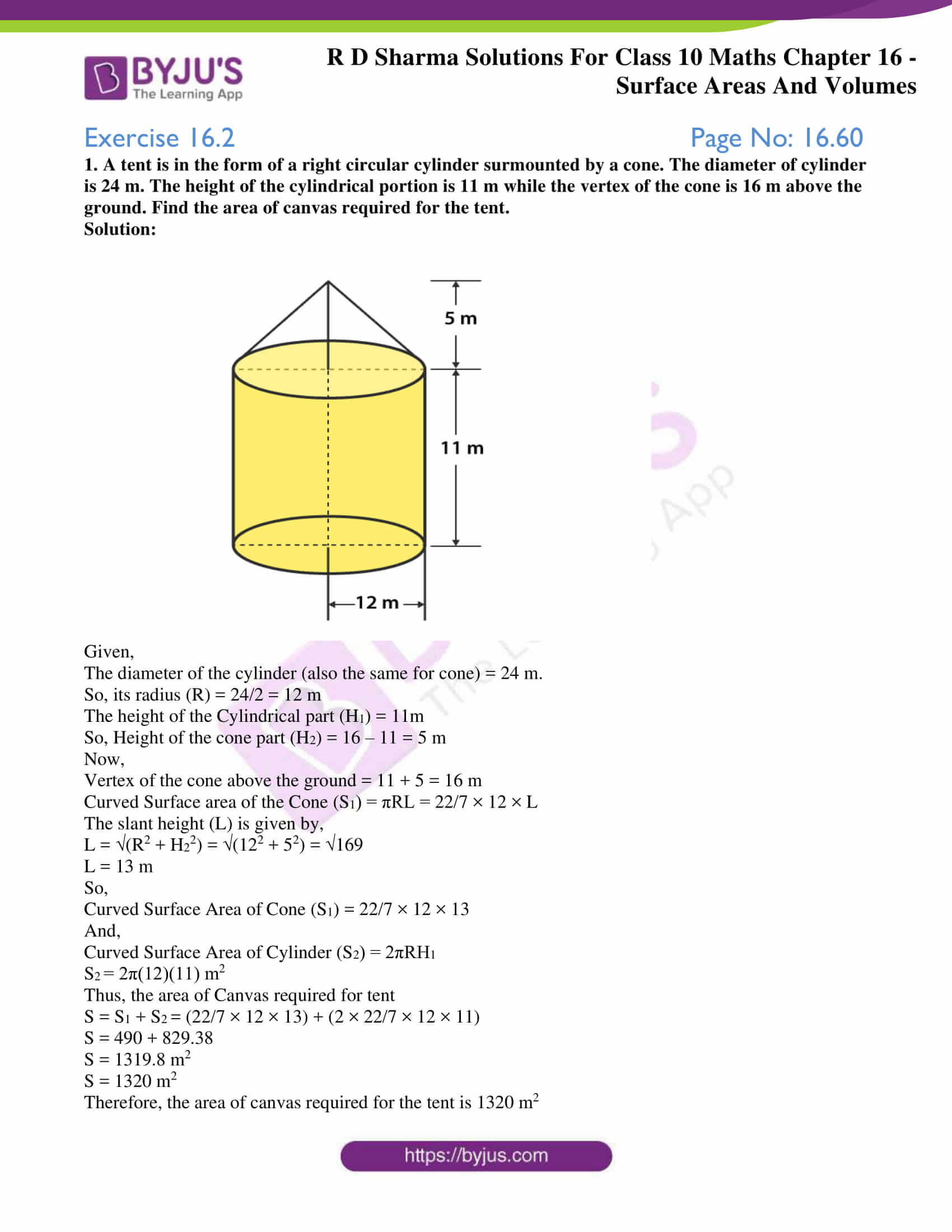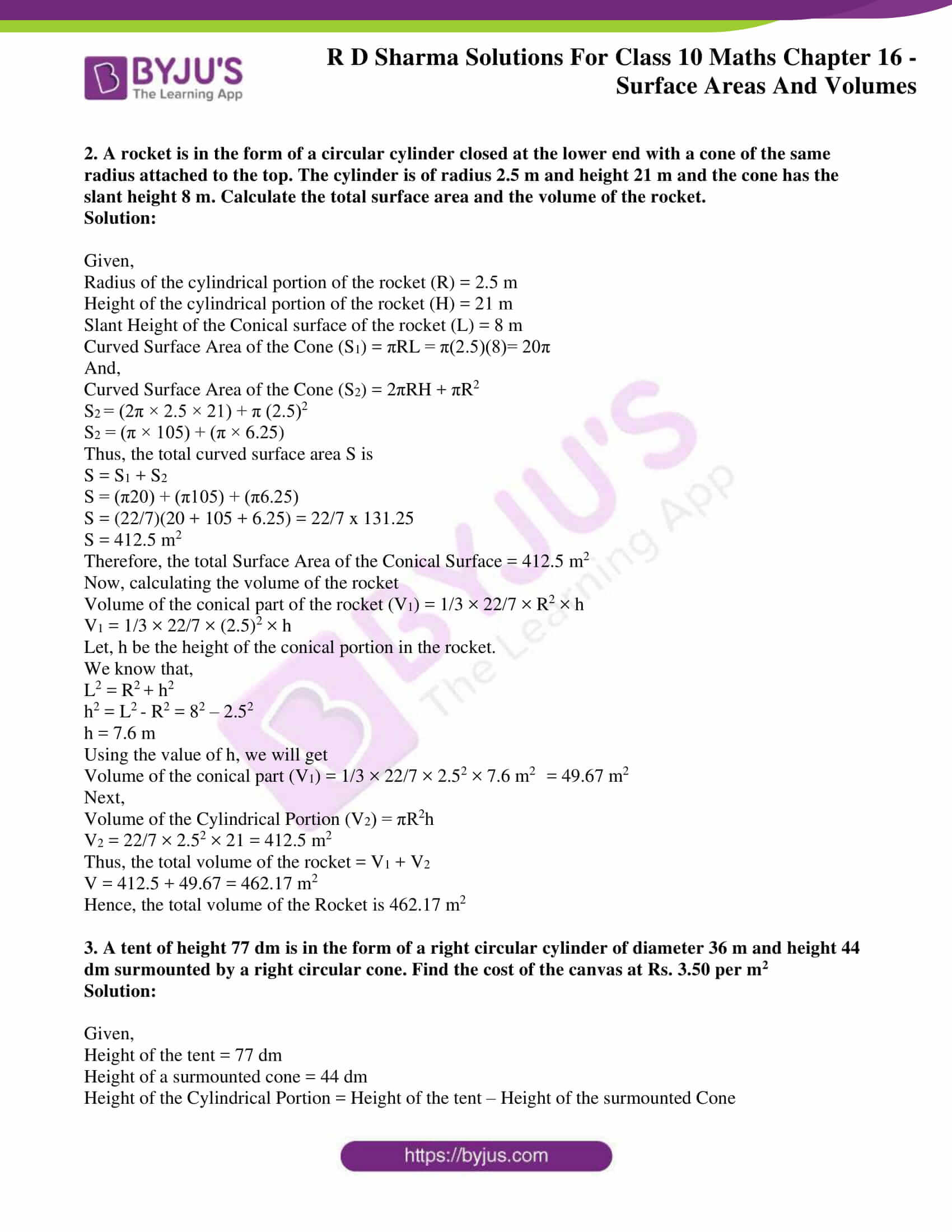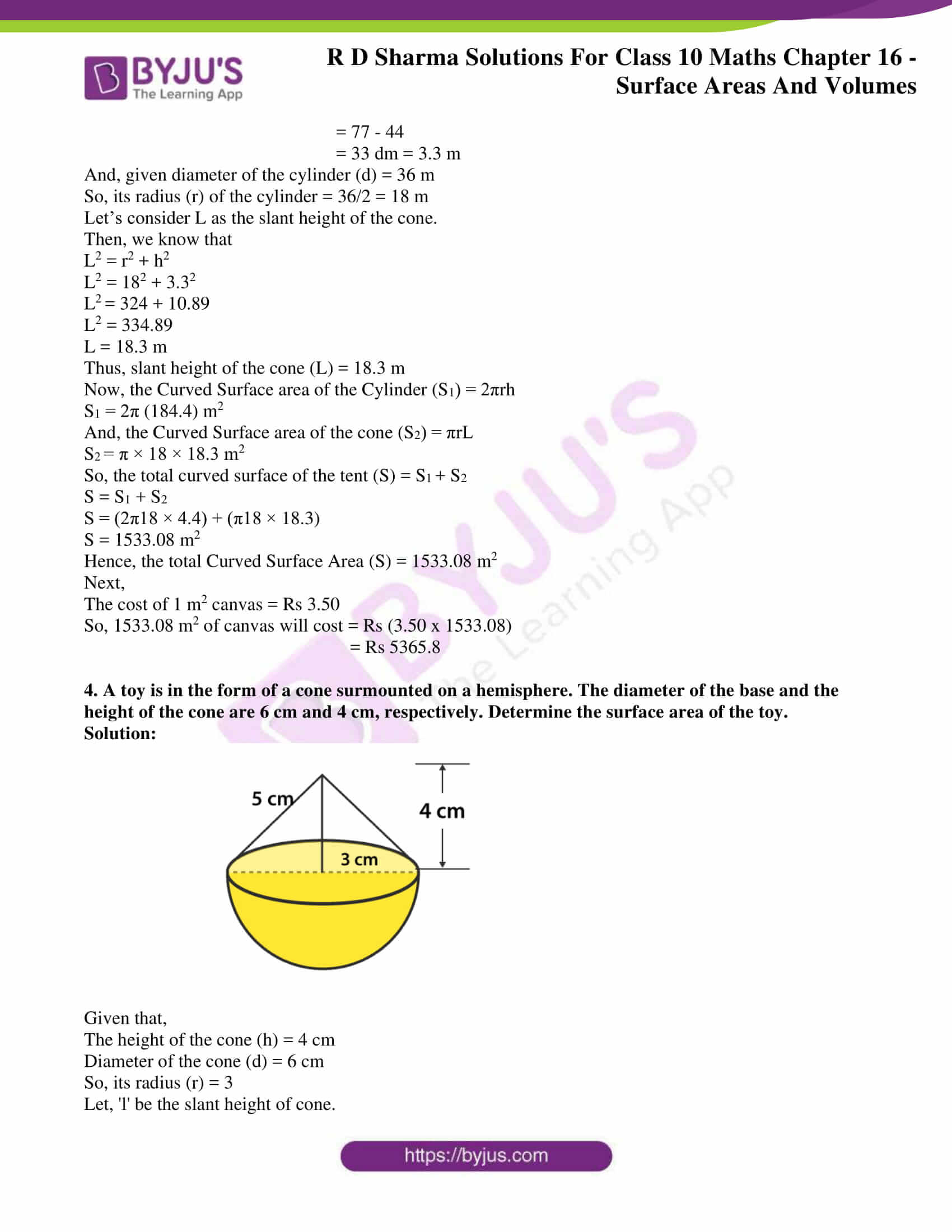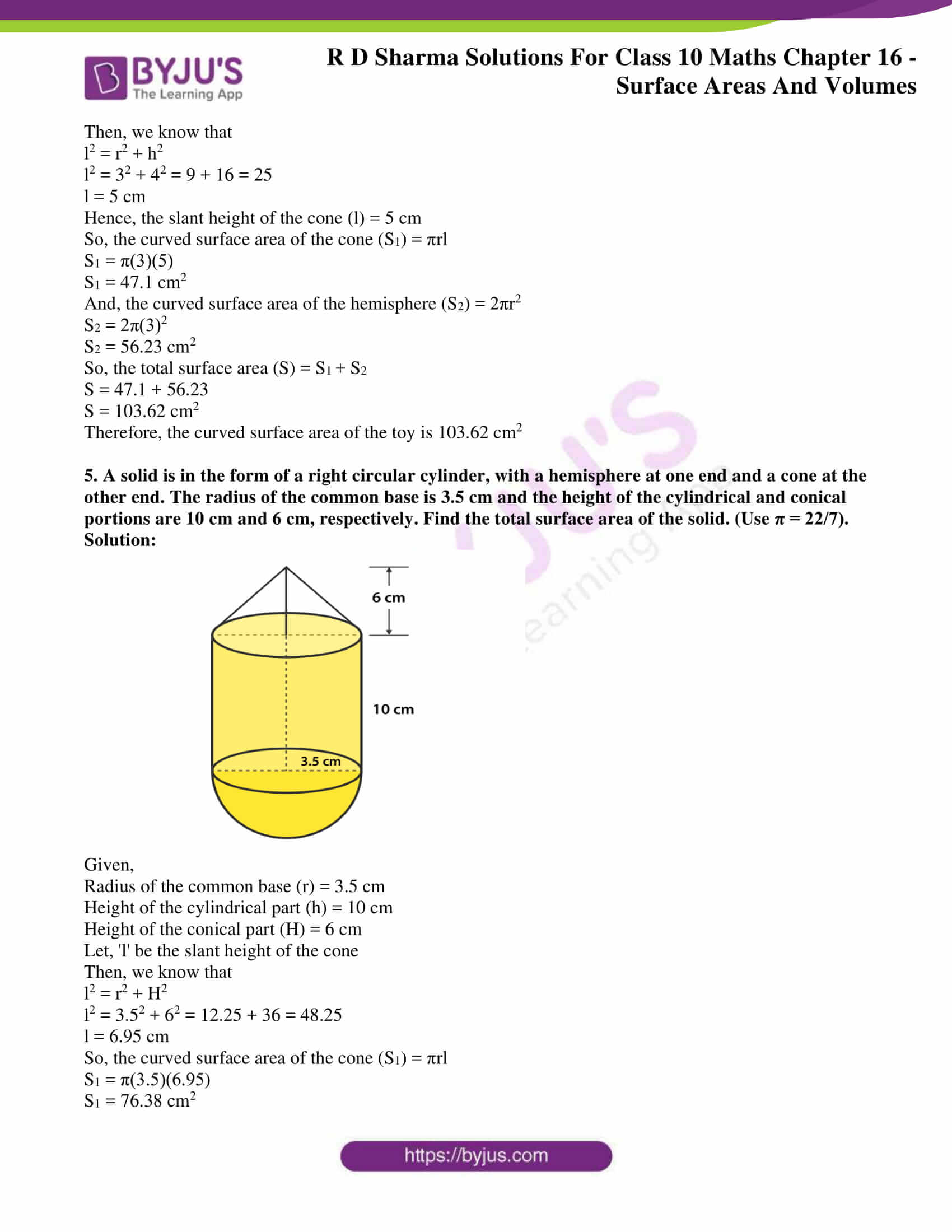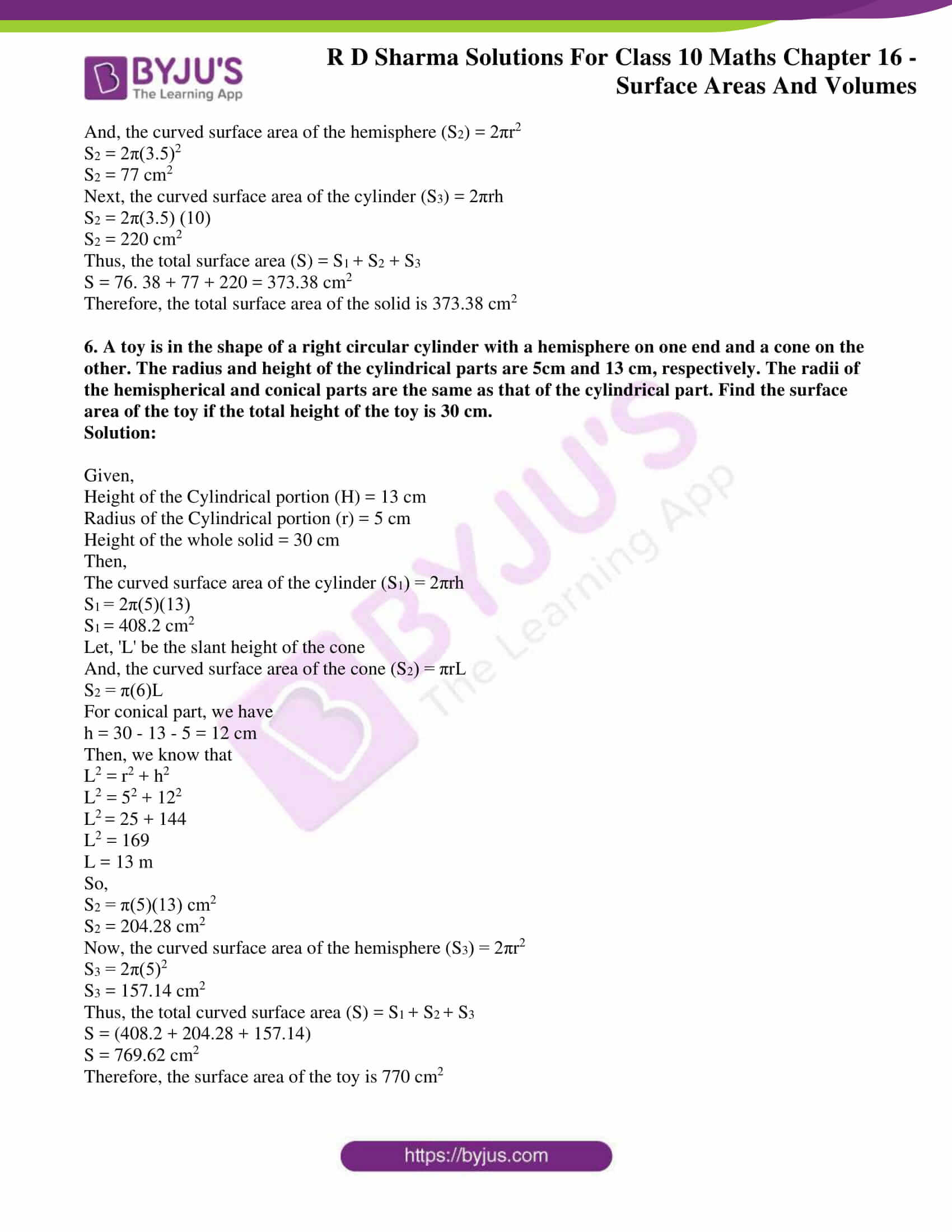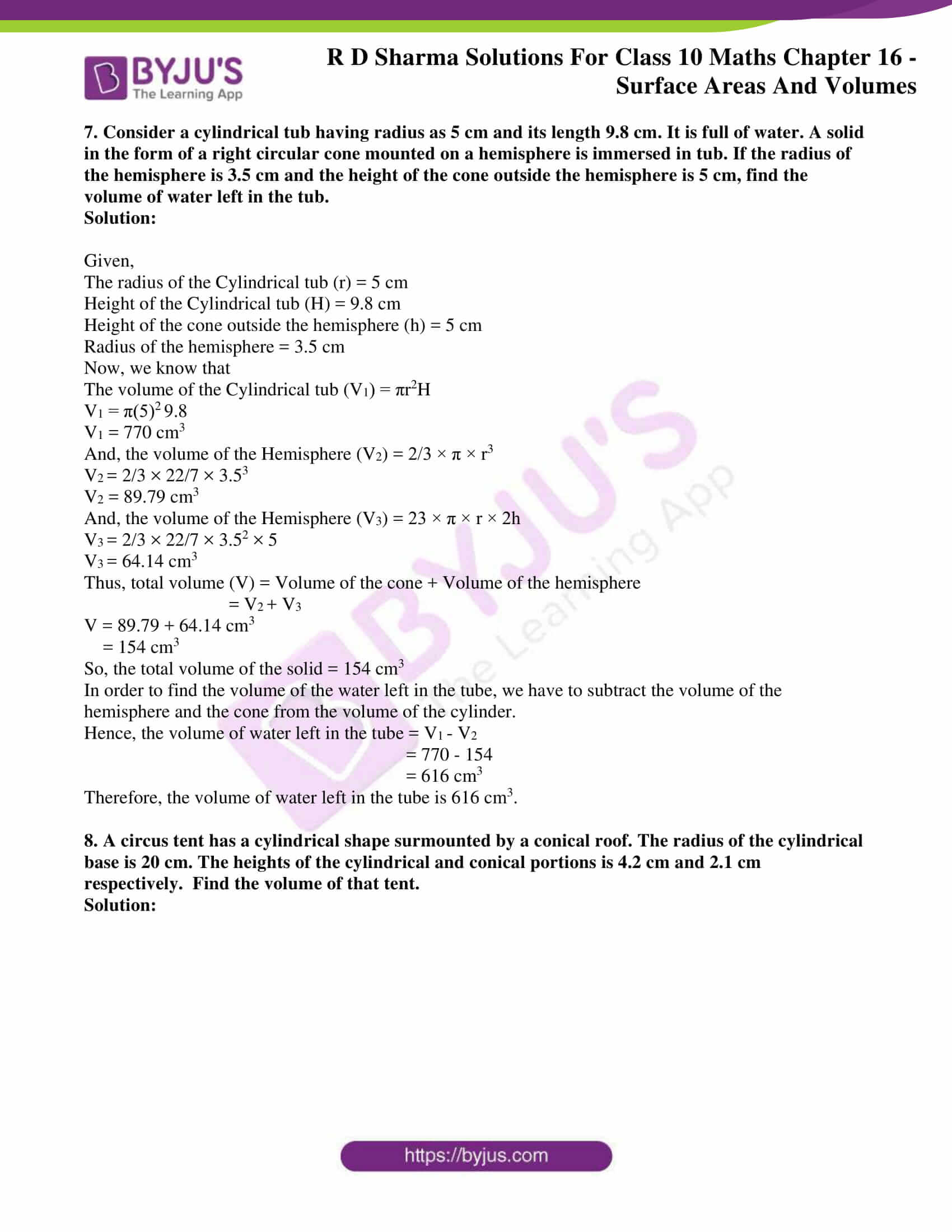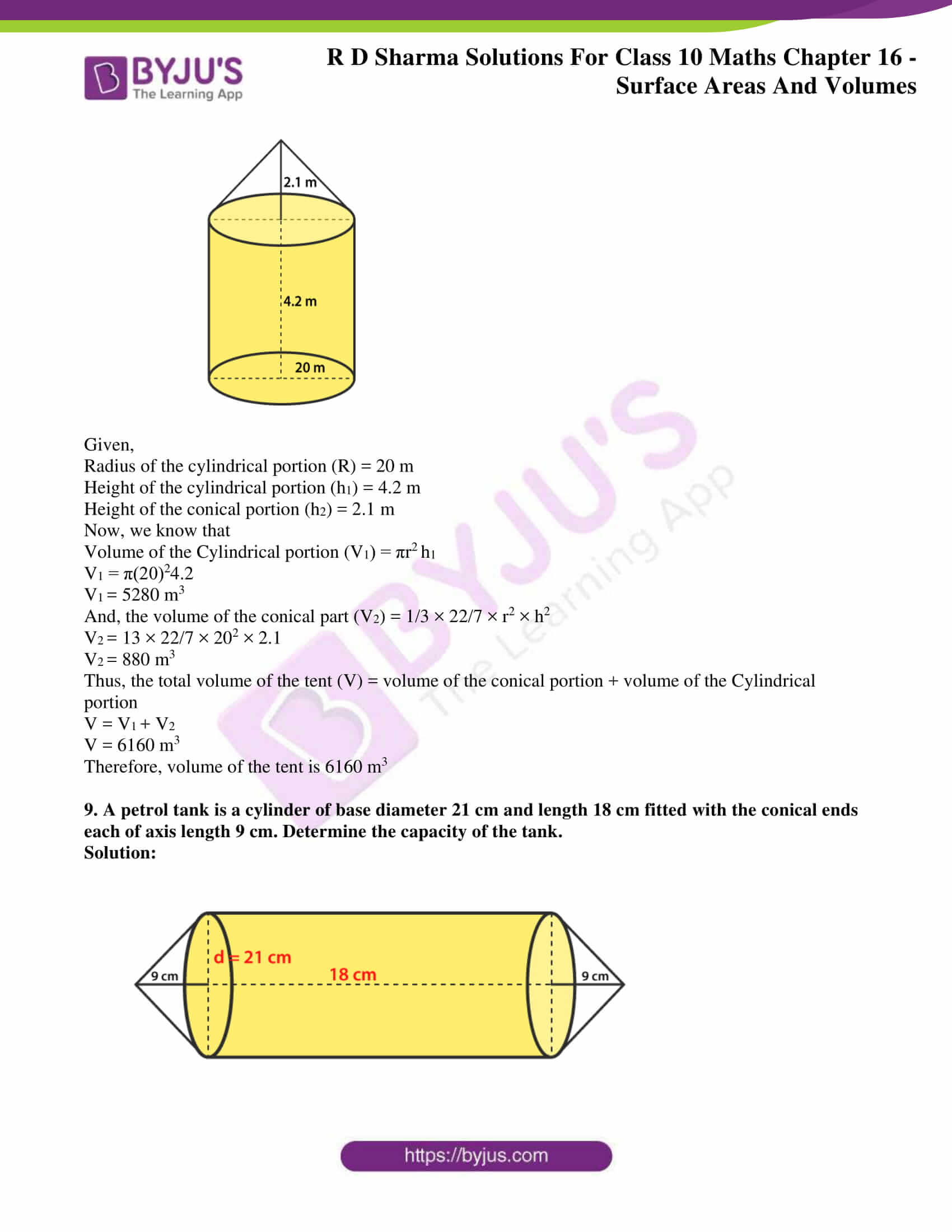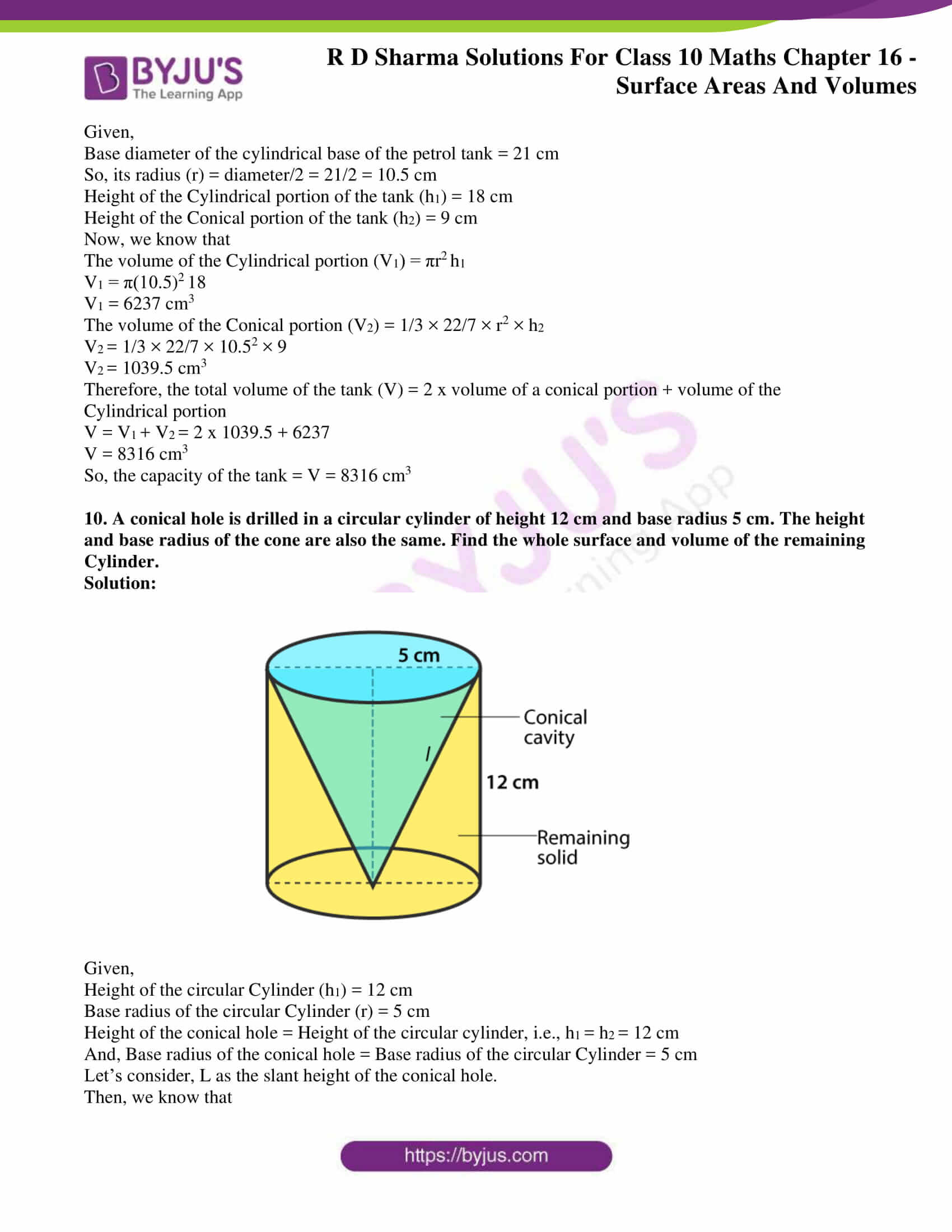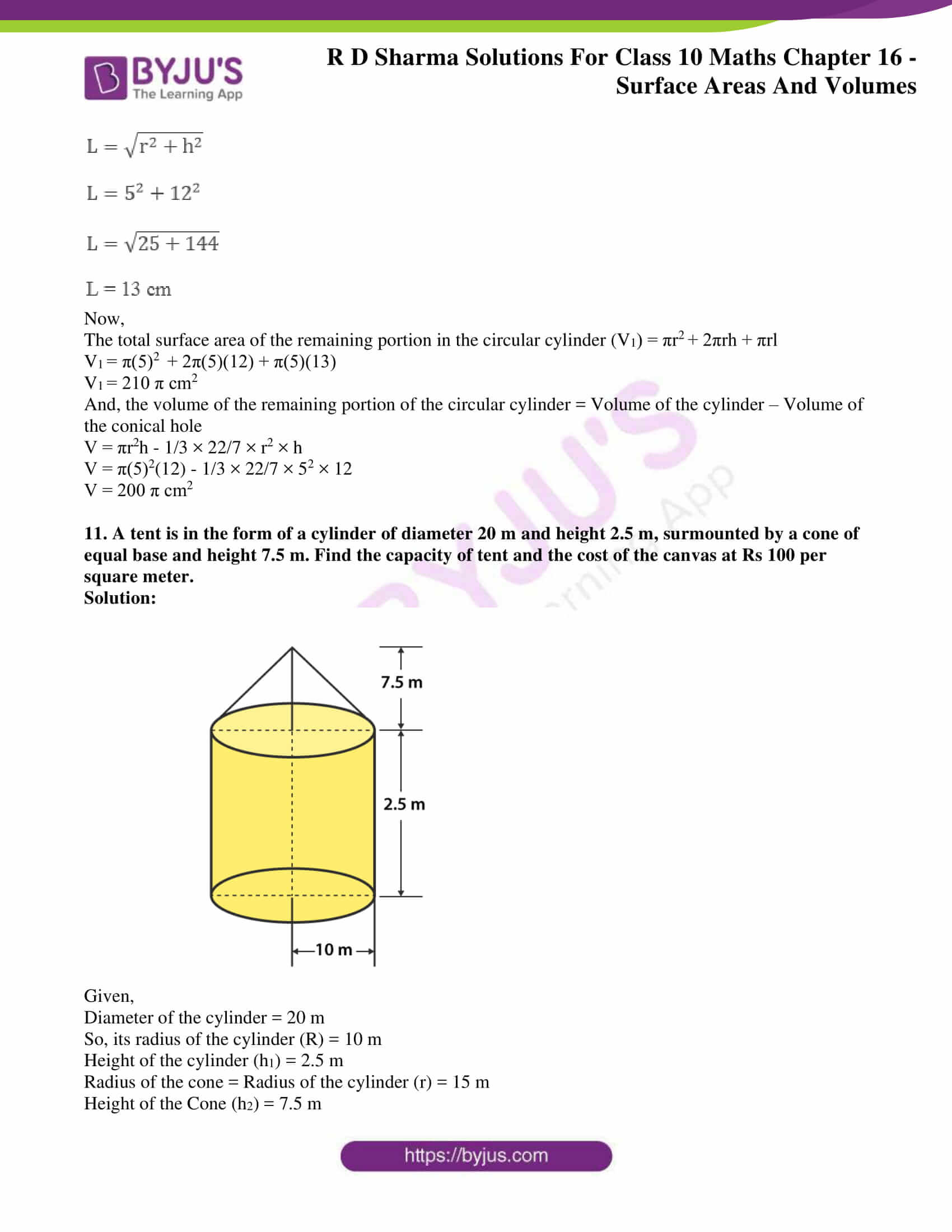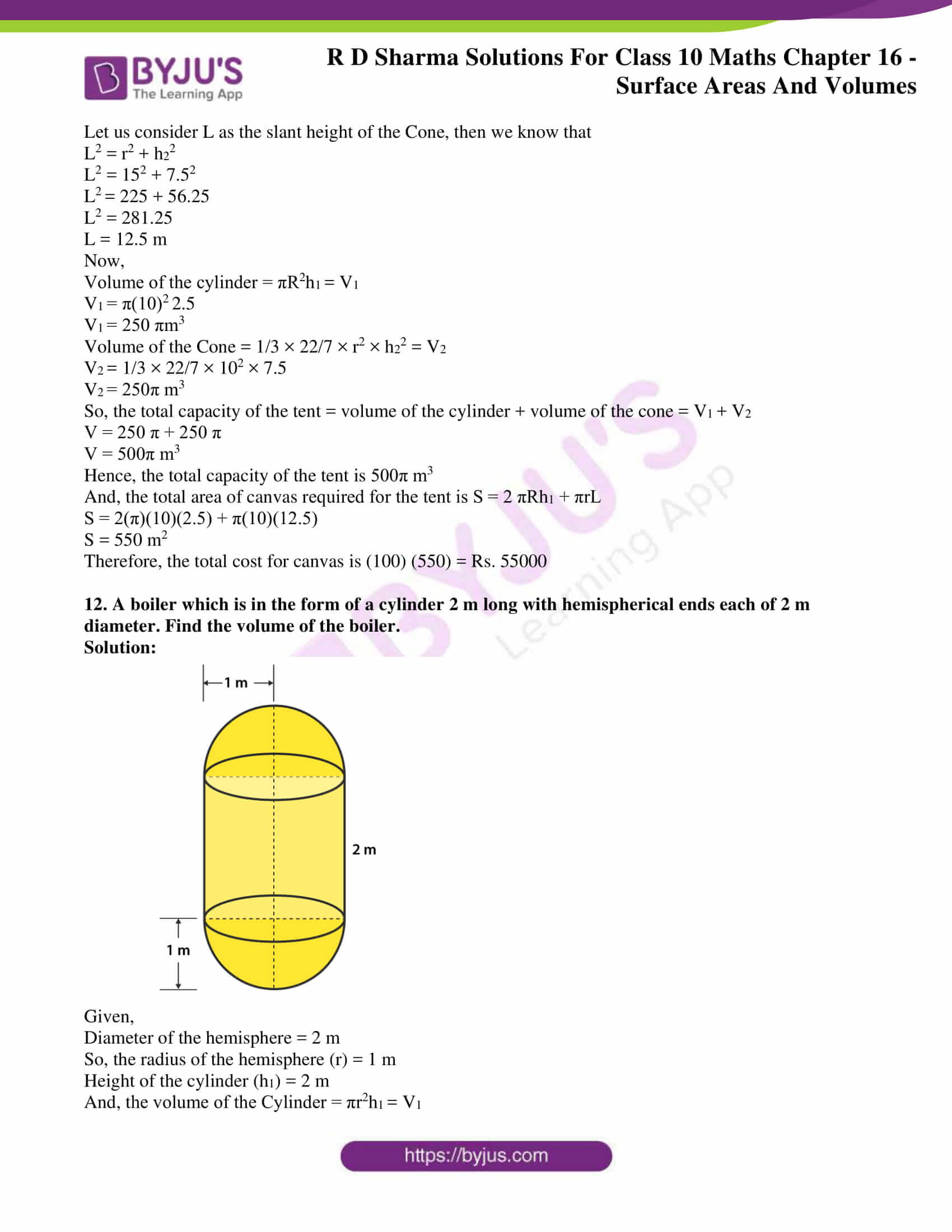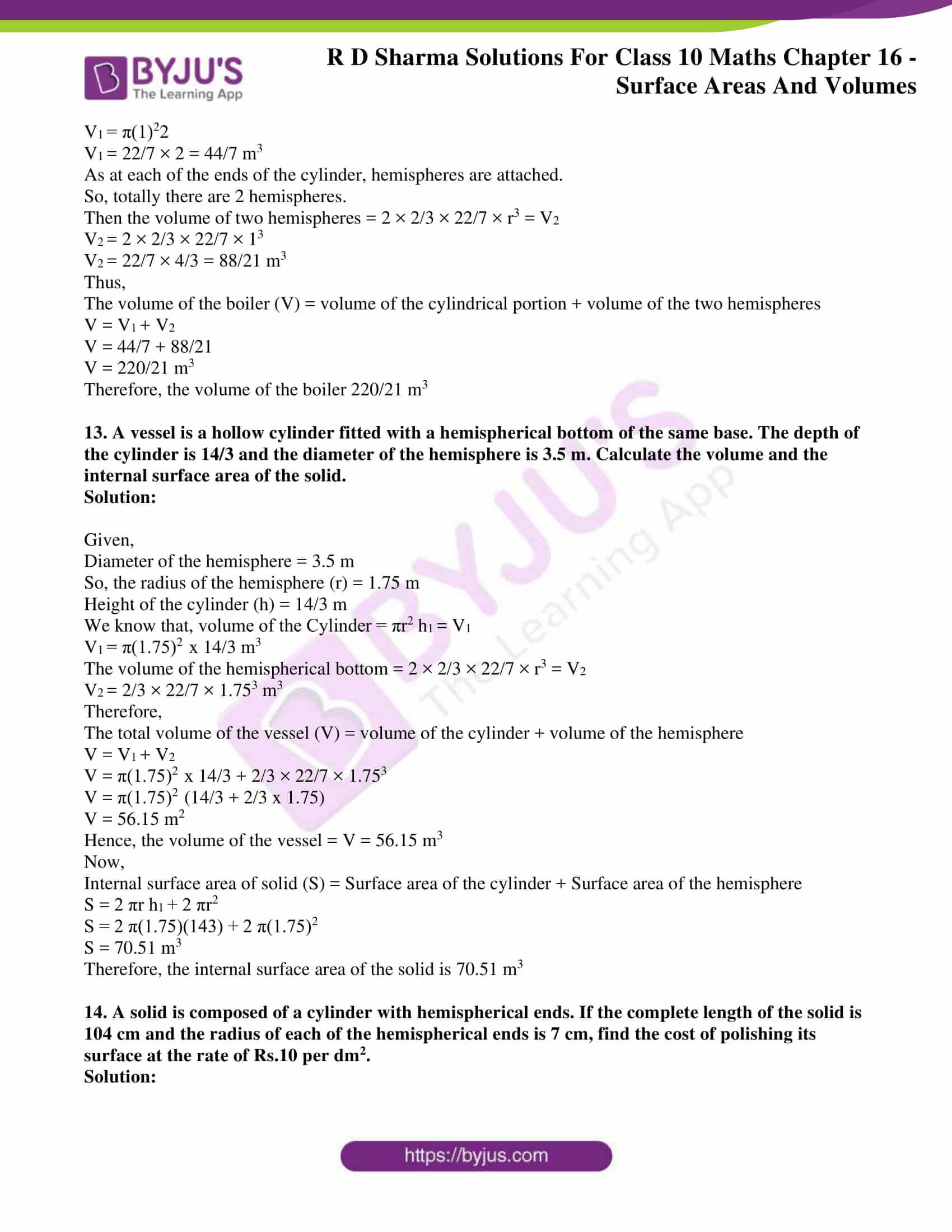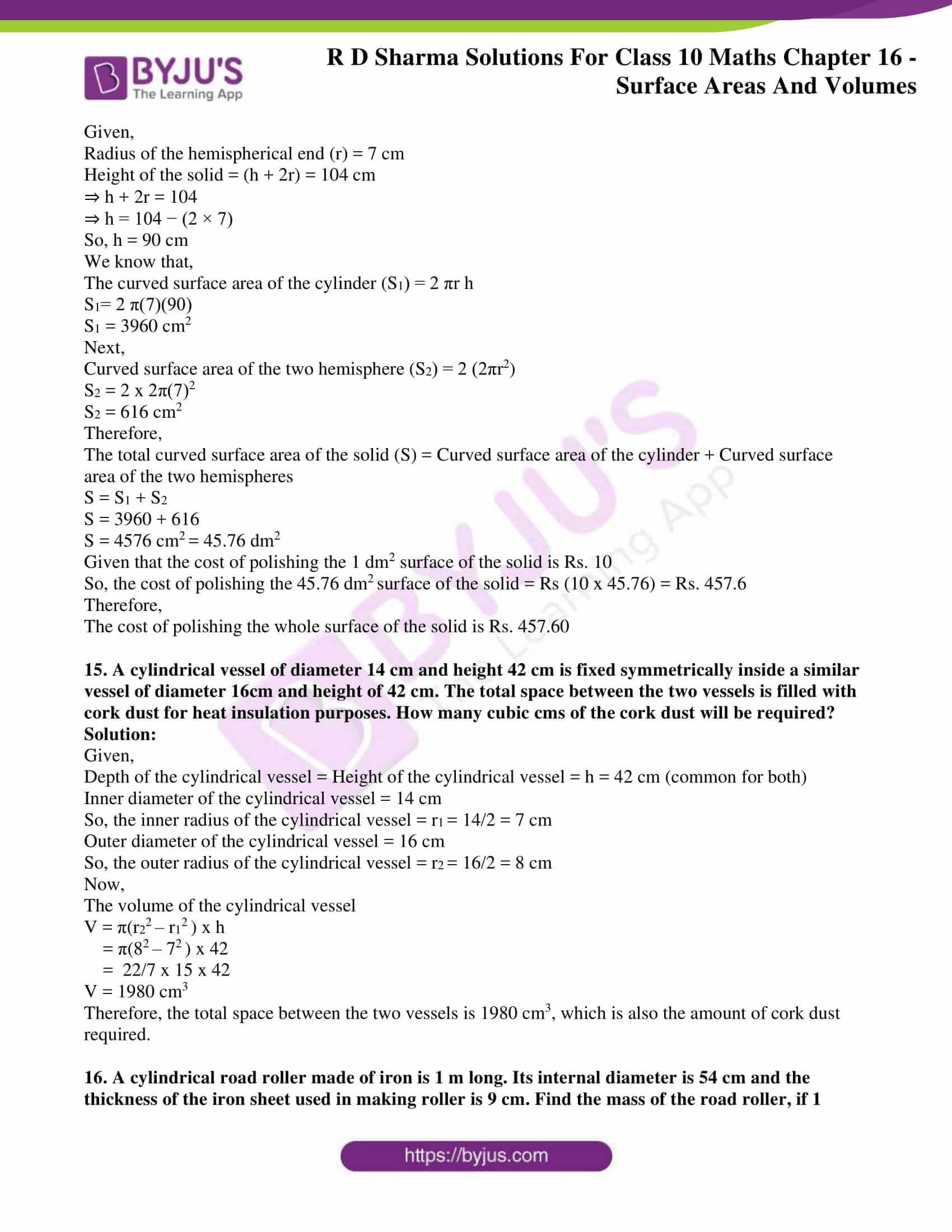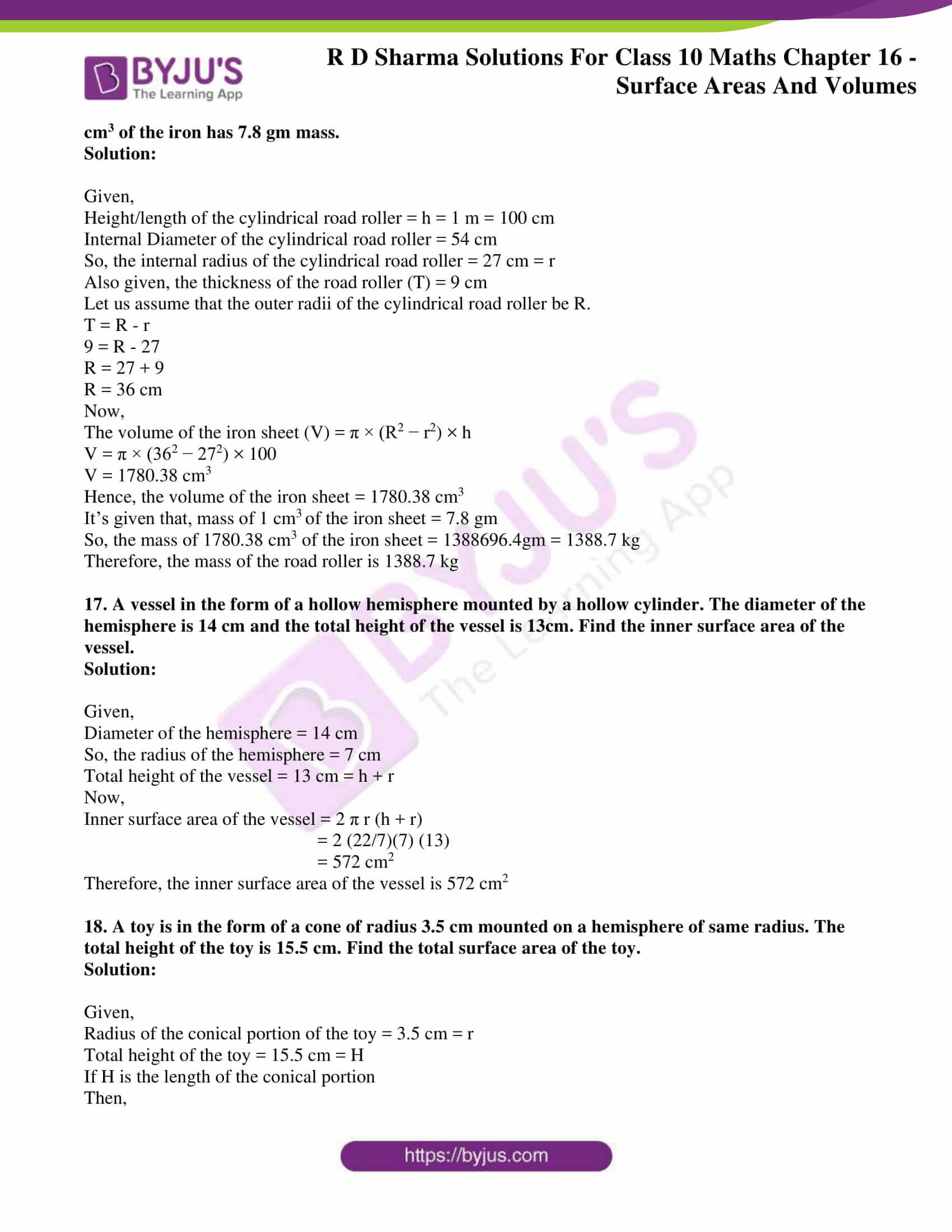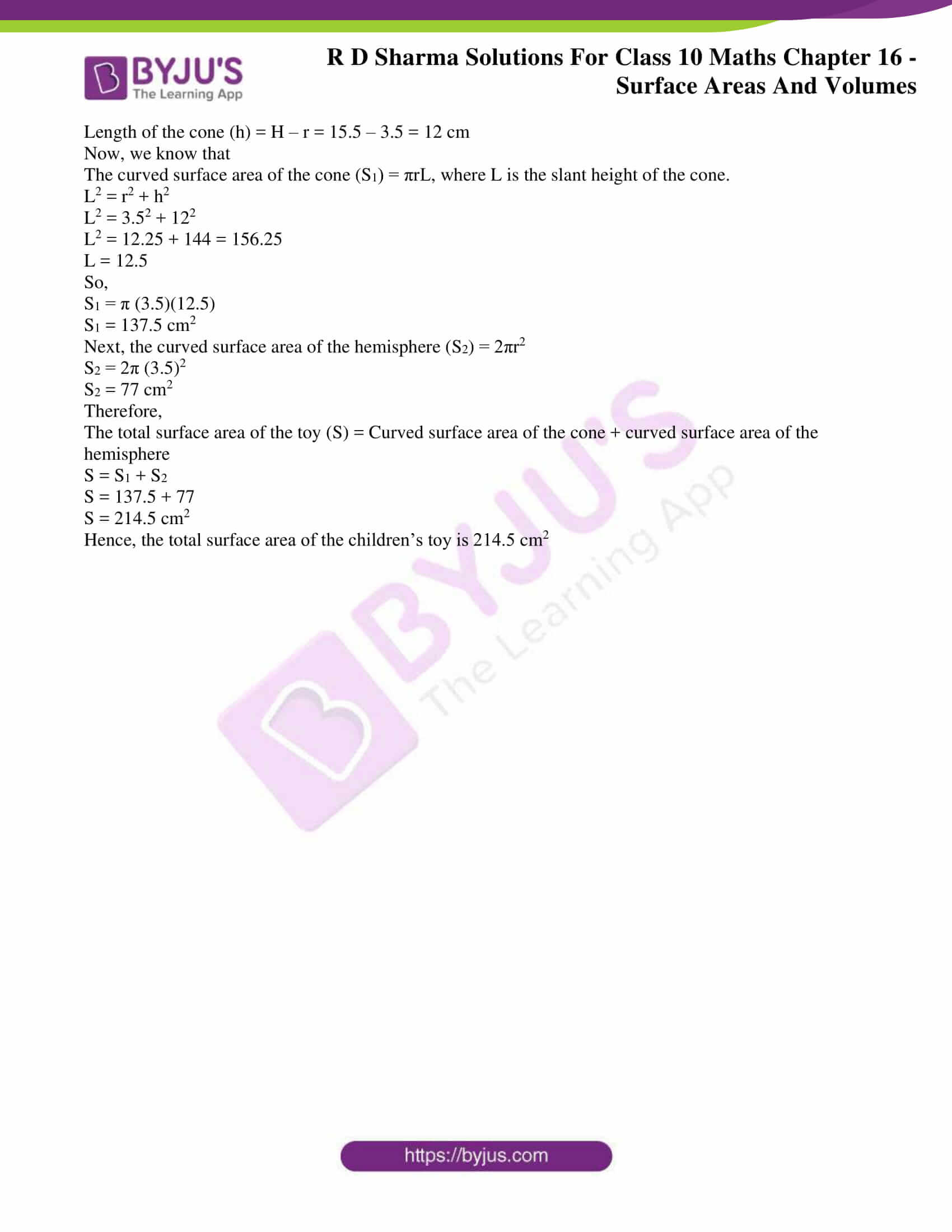### Access RD Sharma Solutions for Class 10 Maths Chapter 16 Surface Areas And Volumes Exercise 16.2

1. A tent is in the form of a right circular cylinder surmounted by a cone. The diameter of cylinder is 24 m. The height of the cylindrical portion is 11 m while the vertex of the cone is 16 m above the ground. Find the area of canvas required for the tent.

Solution: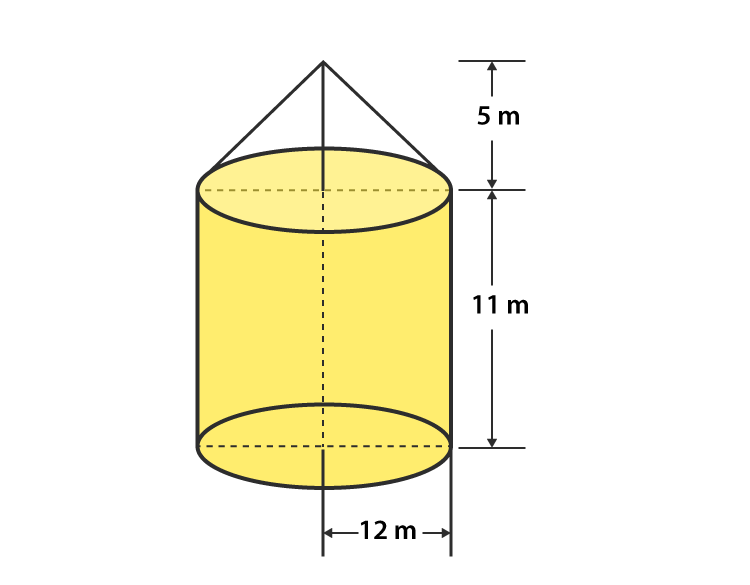Given,

The diameter of the cylinder (also the same for cone) = 24 m.

So, its radius (R) = 24/2 = 12 m

The height of the Cylindrical part (H1) = 11m

So, Height of the cone part (H2) = 16 – 11 = 5 m

Now,

Vertex of the cone above the ground = 11 + 5 = 16 m

Curved Surface area of the Cone (S1) = πRL = 22/7 × 12 × L

The slant height (L) is given by,

L = √(R2 + H22) = √(122 + 52) = √169

L = 13 m

So,

Curved Surface Area of Cone (S1) = 22/7 × 12 × 13

And,

Curved Surface Area of Cylinder (S2) = 2πRH1

S= 2π(12)(11) m2

Thus, the area of Canvas required for tent

S = S1 + S= (22/7 × 12 × 13) + (2 × 22/7 × 12 × 11)

S = 490 + 829.38

S = 1319.8 m2

S = 1320 m2

Therefore, the area of canvas required for the tent is 1320 m2

2. A rocket is in the form of a circular cylinder closed at the lower end with a cone of the same radius attached to the top. The cylinder is of radius 2.5 m and height 21 m and the cone has the slant height 8 m. Calculate the total surface area and the volume of the rocket.

Solution:

Given,

Radius of the cylindrical portion of the rocket (R) = 2.5 m

Height of the cylindrical portion of the rocket (H) = 21 m

Slant Height of the Conical surface of the rocket (L) = 8 m

Curved Surface Area of the Cone (S1) = πRL = π(2.5)(8)= 20π

And,

Curved Surface Area of the Cone (S2) = 2πRH + πR2

S= (2π × 2.5 × 21) + π (2.5)2

S2 = (π × 105) + (π × 6.25)

Thus, the total curved surface area S is

S = S1 + S2

S = (π20) + (π105) + (π6.25)

S = (22/7)(20 + 105 + 6.25) = 22/7 x 131.25

S = 412.5 m2

Therefore, the total Surface Area of the Conical Surface = 412.5 m2

Now, calculating the volume of the rocket

Volume of the conical part of the rocket (V1) = 1/3 × 22/7 × R2 × h

V1 = 1/3 × 22/7 × (2.5)2 × h

Let, h be the height of the conical portion in the rocket.

We know that,

L2 = R+ h2

h2 = L– R2 = 82 – 2.52

h = 7.6 m

Using the value of h, we will get

Volume of the conical part (V1) = 1/3 × 22/7 × 2.52 × 7.6 m2   = 49.67 m2

Next,

Volume of the Cylindrical Portion (V2) = πR2h

V2 = 22/7 × 2.52 × 21 = 412.5 m2

Thus, the total volume of the rocket = V1 + V2

V = 412.5 + 49.67 = 462.17 m2

Hence, the total volume of the Rocket is 462.17 m

3. A tent of height 77 dm is in the form of a right circular cylinder of diameter 36 m and height 44 dm surmounted by a right circular cone. Find the cost of the canvas at Rs. 3.50 per m2

Solution:

Given,

Height of the tent = 77 dm

Height of a surmounted cone = 44 dm

Height of the Cylindrical Portion = Height of the tent – Height of the surmounted Cone

= 77 – 44

= 33 dm = 3.3 m

And, given diameter of the cylinder (d) = 36 m

So, its radius (r) of the cylinder = 36/2 = 18 m

Let’s consider L as the slant height of the cone.

Then, we know that

L2 = r2 + h2

L2 = 182 + 3.32

L= 324 + 10.89

L2 = 334.89

L = 18.3 m

Thus, slant height of the cone (L) = 18.3 m

Now, the Curved Surface area of the Cylinder (S1) = 2πrh

S1 = 2π (184.4) m

And, the Curved Surface area of the cone (S2) = πrL

S= π × 18 × 18.3 m

So, the total curved surface of the tent (S) = S+ S2

S = S1 + S2

S = (2π18 × 4.4) + (π18 × 18.3)

S = 1533.08 m2

Hence, the total Curved Surface Area (S) = 1533.08 m2

Next,

The cost of 1 m2 canvas = Rs 3.50

So, 1533.08 m2 of canvas will cost = Rs (3.50 x 1533.08)

= Rs 5365.8

4. A toy is in the form of a cone surmounted on a hemisphere. The diameter of the base and the height of the cone are 6 cm and 4 cm, respectively. Determine the surface area of the toy.

Solution: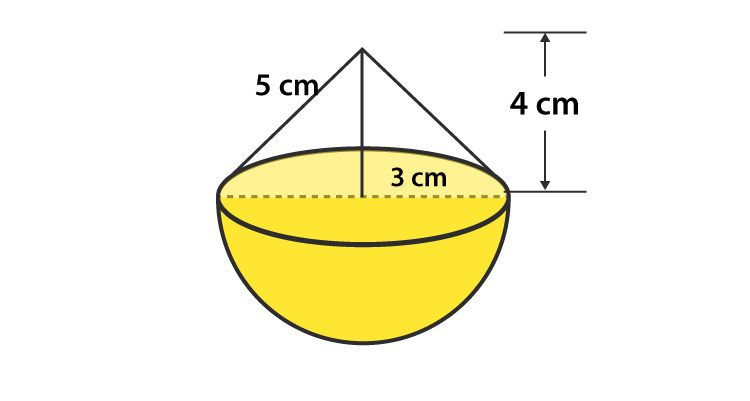Given that,

The height of the cone (h) = 4 cm

Diameter of the cone (d) = 6 cm

So, its radius (r) = 3

Let, ‘l’ be the slant height of cone.

Then, we know that

l2 = r2 + h2

l2 = 32 + 42 = 9 + 16 = 25

l = 5 cm

Hence, the slant height of the cone (l) = 5 cm

So, the curved surface area of the cone (S1) = πrl

S1 = π(3)(5)

S1 = 47.1 cm2

And, the curved surface area of the hemisphere (S2) = 2πr2

S2 = 2π(3)2

S2 = 56.23 cm2

So, the total surface area (S) = S+ S2

S = 47.1 + 56.23

S = 103.62 cm2

Therefore, the curved surface area of the toy is 103.62 cm2

5. A solid is in the form of a right circular cylinder, with a hemisphere at one end and a cone at the other end. The radius of the common base is 3.5 cm and the height of the cylindrical and conical portions are 10 cm and 6 cm, respectively. Find the total surface area of the solid. (Use π = 22/7).

Solution: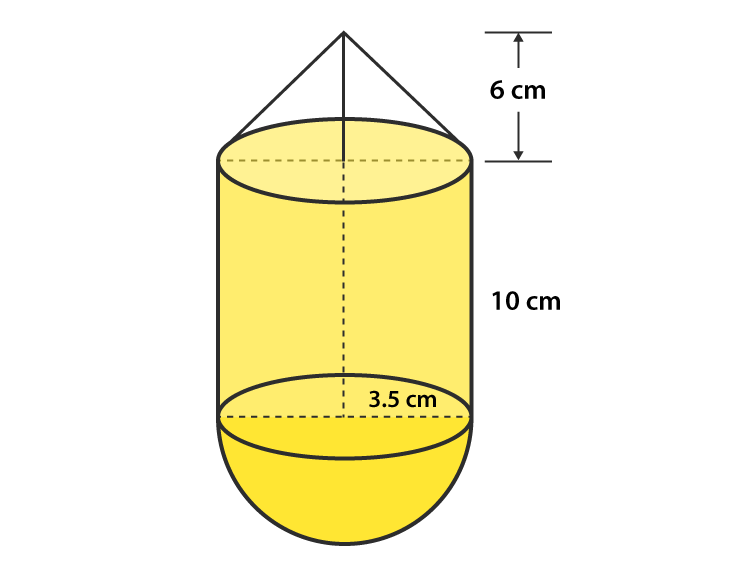Given,

Radius of the common base (r) = 3.5 cm

Height of the cylindrical part (h) = 10 cm

Height of the conical part (H) = 6 cm

Let, ‘l’ be the slant height of the cone

Then, we know that

l2 = r2 + H2

l2 = 3.52 + 62 = 12.25 + 36 = 48.25

l = 6.95 cm

So, the curved surface area of the cone (S1) = πrl

S1 = π(3.5)(6.95)

S1 = 76.38 cm

And, the curved surface area of the hemisphere (S2) = 2πr2

S2 = 2π(3.5)2

S2 = 77 cm2

Next, the curved surface area of the cylinder (S3) = 2πrh

S2 = 2π(3.5) (10)

S2 = 220 cm2

Thus, the total surface area (S) = S+ S2 + S3

S = 76. 38 + 77 + 220 = 373.38 cm2

Therefore, the total surface area of the solid is 373.38 cm2

6. A toy is in the shape of a right circular cylinder with a hemisphere on one end and a cone on the other. The radius and height of the cylindrical parts are 5cm and 13 cm, respectively. The radii of the hemispherical and conical parts are the same as that of the cylindrical part. Find the surface area of the toy if the total height of the toy is 30 cm.

Solution:

Given,

Height of the Cylindrical portion (H) = 13 cm

Radius of the Cylindrical portion (r) = 5 cm

Height of the whole solid = 30 cm

Then,

The curved surface area of the cylinder (S1) = 2πrh

S= 2π(5)(13)

S= 408.2 cm2

Let, ‘L’ be the slant height of the cone

And, the curved surface area of the cone (S2) = πrL

S2 = π(6)L

For conical part, we have

h = 30 – 13 – 5 = 12 cm

Then, we know that

L2 = r2 + h2

L2 = 52 + 122

L= 25 + 144

L2 = 169

L = 13 m

So,

S2 = π(5)(13) cm2

S2 = 204.28 cm2

Now, the curved surface area of the hemisphere (S3) = 2πr2

S3 = 2π(5)2

S3 = 157.14 cm2

Thus, the total curved surface area (S) = S+ S+ S3

S = (408.2 + 204.28 + 157.14)

S = 769.62 cm2

Therefore, the surface area of the toy is 770 cm2

7. Consider a cylindrical tub having radius as 5 cm and its length 9.8 cm. It is full of water. A solid in the form of a right circular cone mounted on a hemisphere is immersed in tub. If the radius of the hemisphere is 3.5 cm and the height of the cone outside the hemisphere is 5 cm, find the volume of water left in the tub.

Solution:

Given,

The radius of the Cylindrical tub (r) = 5 cm

Height of the Cylindrical tub (H) = 9.8 cm

Height of the cone outside the hemisphere (h) = 5 cm

Radius of the hemisphere = 3.5 cm

Now, we know that

The volume of the Cylindrical tub (V1) = πr2H

V1 = π(5)9.8

V1 = 770 cm3

And, the volume of the Hemisphere (V2) = 2/3 × π × r3

V= 2/3 × 22/7 × 3.53

V2 = 89.79 cm3

And, the volume of the Hemisphere (V3) = 23 × π × r × 2h

V= 2/3 × 22/7 × 3.52 × 5

V= 64.14 cm3

Thus, total volume (V) = Volume of the cone + Volume of the hemisphere

= V+ V3

V = 89.79 + 64.14 cm

= 154 cm3

So, the total volume of the solid = 154 cm3

In order to find the volume of the water left in the tube, we have to subtract the volume of the hemisphere and the cone from the volume of the cylinder.

Hence, the volume of water left in the tube = V– V2

= 770 – 154

= 616 cm3

Therefore, the volume of water left in the tube is 616 cm3.

8. A circus tent has a cylindrical shape surmounted by a conical roof. The radius of the cylindrical base is 20 cm. The heights of the cylindrical and conical portions is 4.2 cm and 2.1 cm respectively.  Find the volume of that tent.

Solution: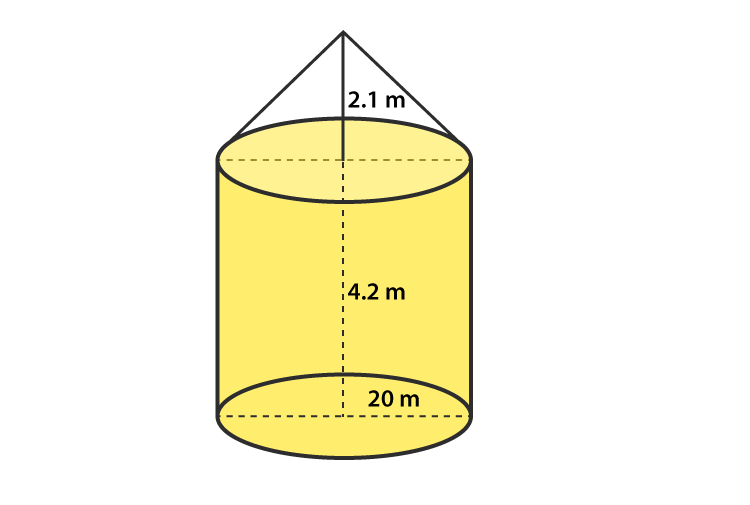Given,

Radius of the cylindrical portion (R) = 20 m

Height of the cylindrical portion (h1) = 4.2 m

Height of the conical portion (h2) = 2.1 m

Now, we know that

Volume of the Cylindrical portion (V1) = πrh1

V1 = π(20)24.2

V= 5280 m3

And, the volume of the conical part (V2) = 1/3 × 22/7 × r2 × h2

V= 13 × 22/7 × 202 × 2.1

V= 880 m3

Thus, the total volume of the tent (V) = volume of the conical portion + volume of the Cylindrical portion

V = V+ V2

V = 6160 m3

Therefore, volume of the tent is 6160 m3

9. A petrol tank is a cylinder of base diameter 21 cm and length 18 cm fitted with the conical ends each of axis length 9 cm. Determine the capacity of the tank.

Solution: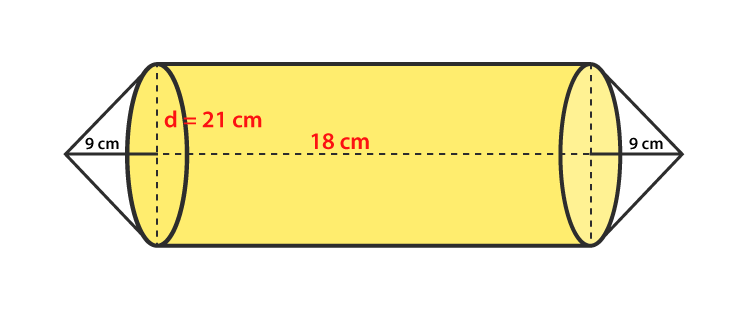Given,

Base diameter of the cylindrical base of the petrol tank = 21 cm

So, its radius (r) = diameter/2 = 21/2 = 10.5 cm

Height of the Cylindrical portion of the tank (h1) = 18 cm

Height of the Conical portion of the tank (h2) = 9 cm

Now, we know that

The volume of the Cylindrical portion (V1) = πrh1

V1 = π(10.5)18

V1 = 6237 cm3

The volume of the Conical portion (V2) = 1/3 × 22/7 × r2 × h2

V= 1/3 × 22/7 × 10.52 × 9

V= 1039.5 cm3

Therefore, the total volume of the tank (V) = 2 x volume of a conical portion + volume of the Cylindrical portion

V = V+ V2 = 2 x 1039.5 + 6237

V = 8316 cm3

So, the capacity of the tank = V = 8316 cm

10. A conical hole is drilled in a circular cylinder of height 12 cm and base radius 5 cm. The height and base radius of the cone are also the same. Find the whole surface and volume of the remaining Cylinder.

Solution: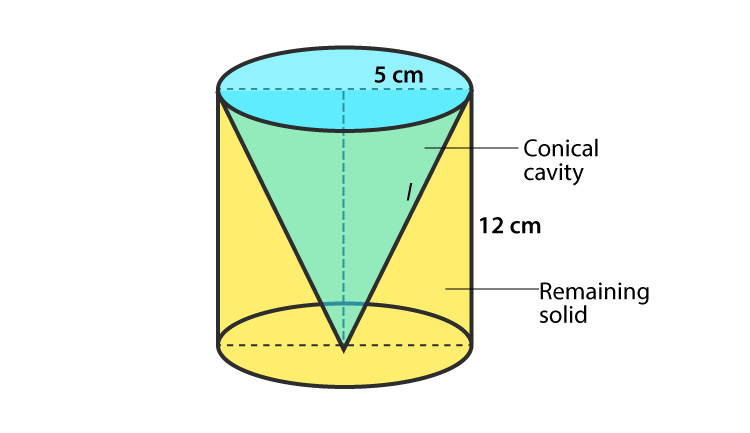Given,

Height of the circular Cylinder (h1) = 12 cm

Base radius of the circular Cylinder (r) = 5 cm

Height of the conical hole = Height of the circular cylinder, i.e., h= h= 12 cm

And, Base radius of the conical hole = Base radius of the circular Cylinder = 5 cm

Let’s consider, L as the slant height of the conical hole.

Then, we know that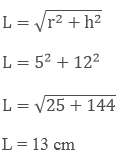Now,

The total surface area of the remaining portion in the circular cylinder (V1) = πr+ 2πrh + πrl

V= π(5) + 2π(5)(12) + π(5)(13)

V= 210 π cm2

And, the volume of the remaining portion of the circular cylinder = Volume of the cylinder – Volume of the conical hole

V = πr2h – 1/3 × 22/7 × r2 × h

V = π(5)2(12) – 1/3 × 22/7 × 52 × 12

V = 200 π cm2

11. A tent is in the form of a cylinder of diameter 20 m and height 2.5 m, surmounted by a cone of equal base and height 7.5 m. Find the capacity of tent and the cost of the canvas at Rs 100 per square meter.

Solution: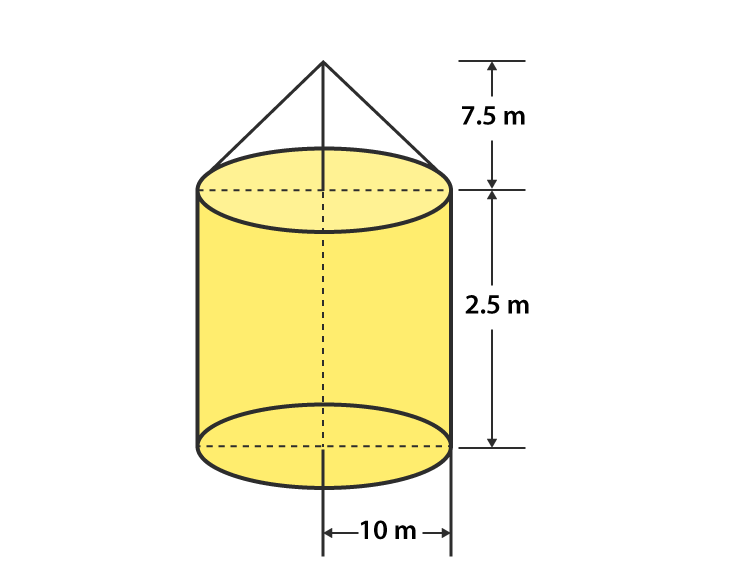Given,

Diameter of the cylinder = 20 m

So, its radius of the cylinder (R) = 10 m

Height of the cylinder (h1) = 2.5 m

Radius of the cone = Radius of the cylinder (r) = 15 m

Height of the Cone (h2) = 7.5 m

Let us consider L as the slant height of the Cone, then we know that

L2 = r2 + h22

L2 = 152 + 7.52

L= 225 + 56.25

L2 = 281.25

L = 12.5 m

Now,

Volume of the cylinder = πR2h= V1

V= π(10)2.5

V= 250 πm3

Volume of the Cone = 1/3 × 22/7 × r2 × h22 = V2

V= 1/3 × 22/7 × 102 × 7.5

V= 250π m3

So, the total capacity of the tent = volume of the cylinder + volume of the cone = V+ V2

V = 250 π + 250 π

V = 500π m3

Hence, the total capacity of the tent is 500π m3

And, the total area of canvas required for the tent is S = 2 πRh1 + πrL

S = 2(π)(10)(2.5) + π(10)(12.5)

S = 550 m2

Therefore, the total cost for canvas is (100) (550) = Rs. 55000

12. A boiler which is in the form of a cylinder 2 m long with hemispherical ends each of 2 m diameter. Find the volume of the boiler.

Solution: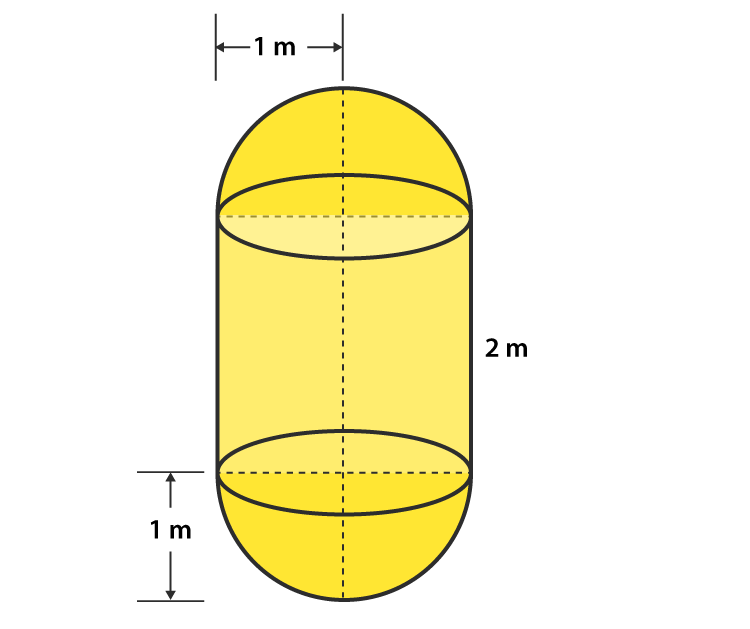Given,

Diameter of the hemisphere = 2 m

So, the radius of the hemisphere (r) = 1 m

Height of the cylinder (h1) = 2 m

And, the volume of the Cylinder = πr2h= V1

V= π(1)22

V= 22/7 × 2 = 44/7 m3

As at each of the ends of the cylinder, hemispheres are attached.

So, totally there are 2 hemispheres.

Then the volume of two hemispheres = 2 × 2/3 × 22/7 × r3 = V2

V= 2 × 2/3 × 22/7 × 13

V= 22/7 × 4/3 = 88/21 m3

Thus,

The volume of the boiler (V) = volume of the cylindrical portion + volume of the two hemispheres

V = V+ V2

V = 44/7 + 88/21

V = 220/21 m3

Therefore, the volume of the boiler 220/21 m3

13. A vessel is a hollow cylinder fitted with a hemispherical bottom of the same base. The depth of the cylinder is 14/3 and the diameter of the hemisphere is 3.5 m. Calculate the volume and the internal surface area of the solid.

Solution:

Given,

Diameter of the hemisphere = 3.5 m

So, the radius of the hemisphere (r) = 1.75 m

Height of the cylinder (h) = 14/3 m

We know that, volume of the Cylinder = πr2 h= V1

V= π(1.75)2 x 14/3 m3

The volume of the hemispherical bottom = 2 × 2/3 × 22/7 × r3 = V2

V= 2/3 × 22/7 × 1.753 m3

Therefore,

The total volume of the vessel (V) = volume of the cylinder + volume of the hemisphere

V = V+ V2

V = π(1.75)2 x 14/3 + 2/3 × 22/7 × 1.753

V = π(1.75)2 (14/3 + 2/3 x 1.75)

V = 56.15 m2

Hence, the volume of the vessel = V = 56.15 m3

Now,

Internal surface area of solid (S) = Surface area of the cylinder + Surface area of the hemisphere

S = 2 πr h+ 2 πr2

S = 2 π(1.75)(143) + 2 π(1.75)2

S = 70.51 m3

Therefore, the internal surface area of the solid is 70.51 m3

14. A solid is composed of a cylinder with hemispherical ends. If the complete length of the solid is 104 cm and the radius of each of the hemispherical ends is 7 cm, find the cost of polishing its surface at the rate of Rs.10 per dm2.

Solution:

Given,

Radius of the hemispherical end (r) = 7 cm

Height of the solid = (h + 2r) = 104 cm

⇒ h + 2r = 104

⇒ h = 104 − (2 × 7)

So, h = 90 cm

We know that,

The curved surface area of the cylinder (S1) = 2 πr h

S1= 2 π(7)(90)

S1 = 3960 cm2

Next,

Curved surface area of the two hemisphere (S2) = 2 (2πr2)

S2 = 2 x 2π(7)2

S2 = 616 cm2

Therefore,

The total curved surface area of the solid (S) = Curved surface area of the cylinder + Curved surface area of the two hemispheres

S = S1 + S2

S = 3960 + 616

S = 4576 cm= 45.76 dm2

Given that the cost of polishing the 1 dm2 surface of the solid is Rs. 10

So, the cost of polishing the 45.76 dmsurface of the solid = Rs (10 x 45.76) = Rs. 457.6

Therefore,

The cost of polishing the whole surface of the solid is Rs. 457.60

15. A cylindrical vessel of diameter 14 cm and height 42 cm is fixed symmetrically inside a similar vessel of diameter 16cm and height of 42 cm. The total space between the two vessels is filled with cork dust for heat insulation purposes. How many cubic cms of the cork dust will be required?

Solution:

Given,

Depth of the cylindrical vessel = Height of the cylindrical vessel = h = 42 cm (common for both)

Inner diameter of the cylindrical vessel = 14 cm

So, the inner radius of the cylindrical vessel = r= 14/2 = 7 cm

Outer diameter of the cylindrical vessel = 16 cm

So, the outer radius of the cylindrical vessel = r= 16/2 = 8 cm

Now,

The volume of the cylindrical vessel

V = π(r22 – r12 ) x h

= π(82 – 72 ) x 42

= 22/7 x 15 x 42

V = 1980 cm3

Therefore, the total space between the two vessels is 1980 cm3, which is also the amount of cork dust required.

16. A cylindrical road roller made of iron is 1 m long. Its internal diameter is 54 cm and the thickness of the iron sheet used in making roller is 9 cm. Find the mass of the road roller, if 1 cm3 of the iron has 7.8 gm mass.

Solution:

Given,

Height/length of the cylindrical road roller = h = 1 m = 100 cm

Internal Diameter of the cylindrical road roller = 54 cm

So, the internal radius of the cylindrical road roller = 27 cm = r

Also given, the thickness of the road roller (T) = 9 cm

Let us assume that the outer radii of the cylindrical road roller be R.

T = R – r

9 = R – 27

R = 27 + 9

R = 36 cm

Now,

The volume of the iron sheet (V) = π × (R2 − r2) × h

V = π × (362 − 272) × 100

V = 1780.38 cm3

Hence, the volume of the iron sheet = 1780.38 cm3

It’s given that, mass of 1 cmof the iron sheet = 7.8 gm

So, the mass of 1780.38 cm3 of the iron sheet = 1388696.4gm = 1388.7 kg

Therefore, the mass of the road roller is 1388.7 kg

17. A vessel in the form of a hollow hemisphere mounted by a hollow cylinder. The diameter of the hemisphere is 14 cm and the total height of the vessel is 13cm. Find the inner surface area of the vessel.

Solution:

Given,

Diameter of the hemisphere = 14 cm

So, the radius of the hemisphere = 7 cm

Total height of the vessel = 13 cm = h + r

Now,

Inner surface area of the vessel = 2 π r (h + r)

= 2 (22/7)(7) (13)

= 572 cm

Therefore, the inner surface area of the vessel is 572 cm2

18. A toy is in the form of a cone of radius 3.5 cm mounted on a hemisphere of same radius. The total height of the toy is 15.5 cm. Find the total surface area of the toy.

Solution:

Given,

Radius of the conical portion of the toy = 3.5 cm = r

Total height of the toy = 15.5 cm = H

If H is the length of the conical portion

Then,

Length of the cone (h) = H – r = 15.5 – 3.5 = 12 cm

Now, we know that

The curved surface area of the cone (S1) = πrL, where L is the slant height of the cone.

L2 = r2 + h2

L2 = 3.52 + 122

L2 = 12.25 + 144 = 156.25

L = 12.5

So,

S1 = π (3.5)(12.5)

S1 = 137.5 cm2

Next, the curved surface area of the hemisphere (S2) = 2πr2

S2 = 2π (3.5)2

S2 = 77 cm2

Therefore,

The total surface area of the toy (S) = Curved surface area of the cone + curved surface area of the hemisphere

S = S1 + S2

S = 137.5 + 77

S = 214.5 cm2

Hence, the total surface area of the children’s toy is 214.5 cm